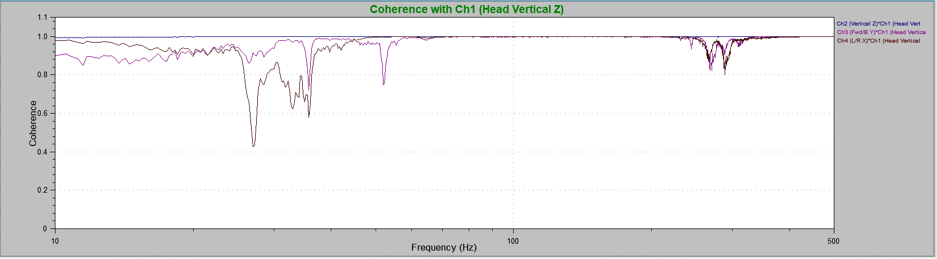# Coherence

January 3, 2019

Coherence is a graph option for the random and shock test modules when the Analyzer key is turned on. The coherence graph plots the correlation calculation in the frequency domain. Two signals are considered coherent if they have a constant phase difference and the same frequency and waveform.

The coherence plot determines how the response signal is related to the drive signal. Values for coherence will be between 0 and 1. A value of 0 indicates there is no relationship between the two signals and a value of 1 indicates there is a perfect relationship between the two signals.

### Uses for Coherence Plots

Coherence plots are helpful to test engineers in several scenarios.

First, they are used to determine the quality of the control unit. A coherence value close to 1 indicates a perfect relationship between the input and output signals and thereby demonstrates that the controller is capable of controlling the test. A coherence value close to 0 indicates a very low quality of control. Low quality of control may occur if the controller is not performing correctly or if there are issues with another part of the testing system such as a damaged cable.

Coherence plots are also helpful when used in conjunction with multi-axis systems. In this case, the plots will demonstrate how the vibrations in one axis affect the vibrations in another axis.

Consider the following test results taken from a vibrating mass of aluminum supported by a thin steel rod. A tri-axis accelerometer was used to measure vibrations at the end of the mass (Figure 2.4).

As the beam vibrates in the vertical plane (z-axis), one would not expect much vibration in the horizontal planes (x- and y-axis). However, at certain frequencies, the energy of the vertical vibrations excites vibrations in the other planes (Figure 2.5). Consequently, the coherence values differ because there no longer is a constant phase difference. A coherence plot is helpful during multi-axis testing to identify the frequencies where the vibrations in one axis are affecting the vibrations in another axis.Figure 2.5. Coherence plot for aluminum mass vibrations in the x-axis (Ch.3) and y-axis (Ch.4) compared to shaker head vibrations (vertical; Ch.1). At several frequencies, the x- and y-axis signals do not correlate with the vertical axis vibrations.

### Conclusion

The coherence plot displays the relationship between the response signal and the drive signal for the frequency domain. Although coherence plots are not commonly used with the single-axis systems, they can be used to ensure that nothing out of ordinary is occurring and to determine the quality of control. In multi-axis systems, the coherence plot can be used to identify how the vibrations in one axis affect the vibrations in another axis.